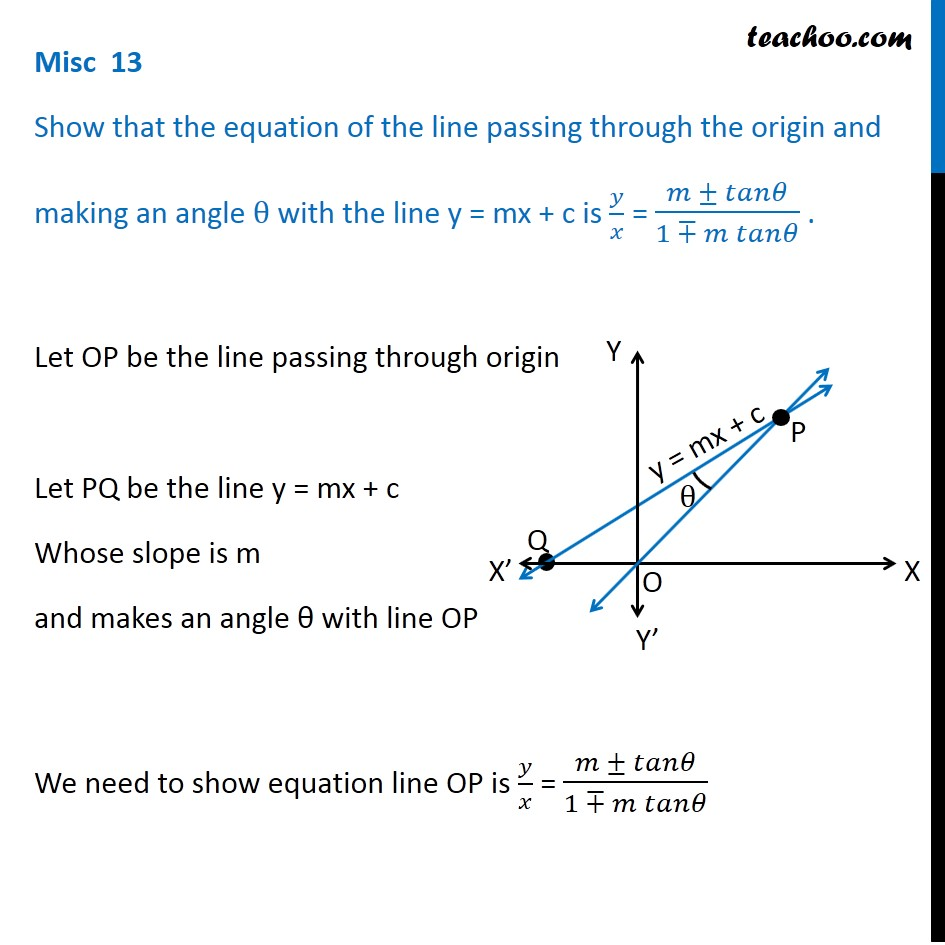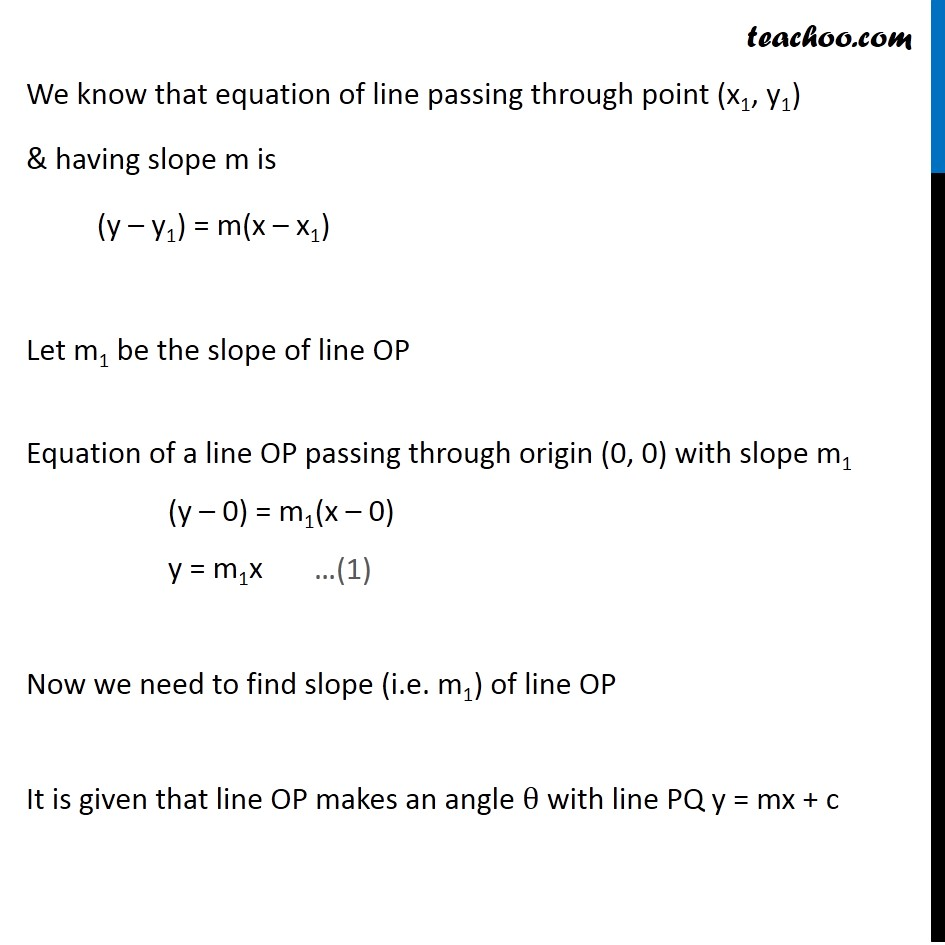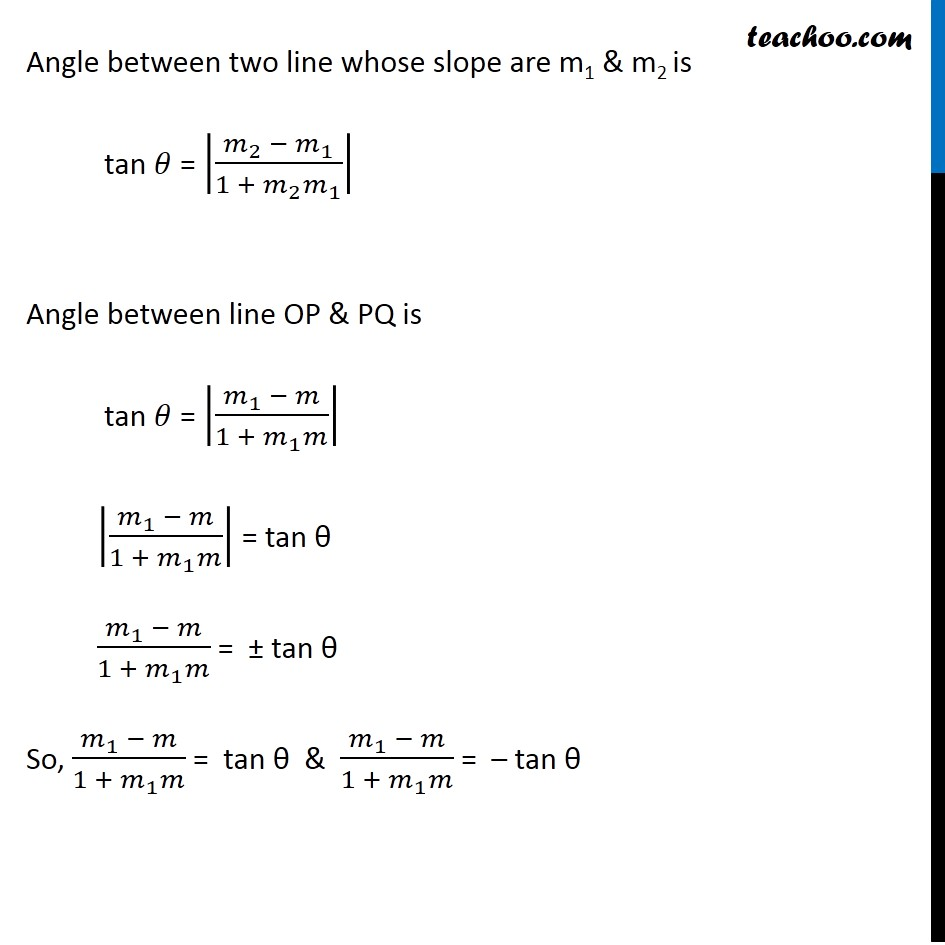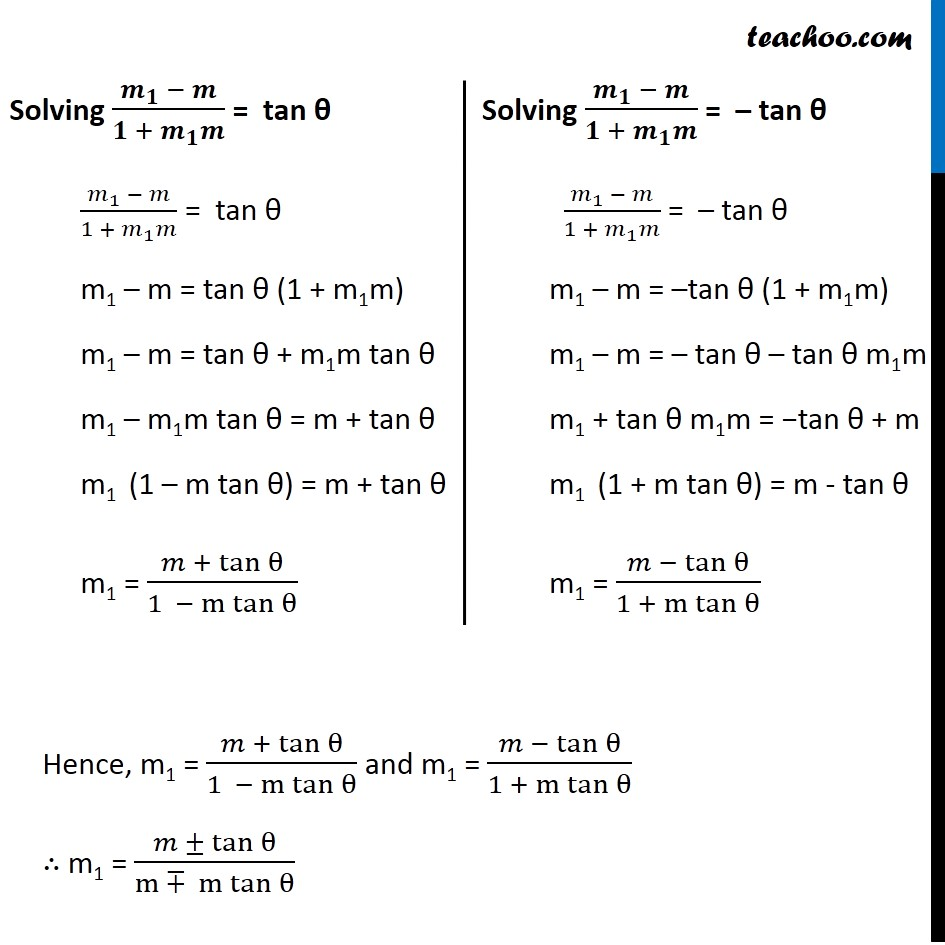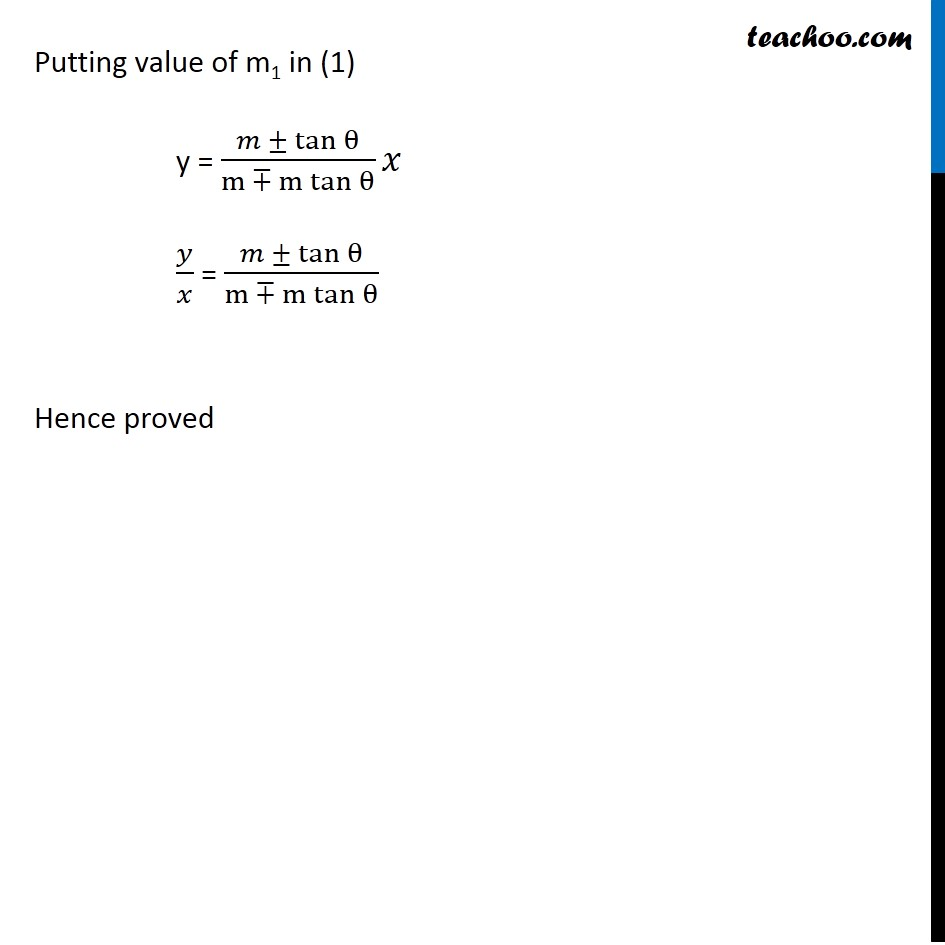1. Chapter 10 Class 11 Straight Lines (Term 1)
2. Serial order wise
3. Miscellaneous

Transcript

Misc 13 Show that the equation of the line passing through the origin and making an angle θ with the line y = mx + c is 𝑦/𝑥 = (𝑚 ± 𝑡𝑎𝑛𝜃)/(1 ∓ 𝑚 𝑡𝑎𝑛𝜃) . Let OP be the line passing through origin Let PQ be the line y = mx + c Whose slope is m and makes an angle θ with line OP We need to show equation line OP is 𝑦/𝑥 = (𝑚 ± 𝑡𝑎𝑛𝜃)/(1 ∓ 𝑚 𝑡𝑎𝑛𝜃) We know that equation of line passing through point (x1, y1) & having slope m is (y – y1) = m(x – x1) Let m1 be the slope of line OP Equation of a line OP passing through origin (0, 0) with slope m1 (y – 0) = m1(x – 0) y = m1x Now we need to find slope (i.e. m1) of line OP It is given that line OP makes an angle θ with line PQ y = mx + c We know that equation of line passing through point (x1, y1) & having slope m is (y – y1) = m(x – x1) Let m1 be the slope of line OP Equation of a line OP passing through origin (0, 0) with slope m1 (y – 0) = m1(x – 0) y = m1x Now we need to find slope (i.e. m1) of line OP It is given that line OP makes an angle θ with line PQ y = mx + c Angle between two line whose slope are m1 & m2 is tan 𝜃 = |(𝑚_2 − 𝑚_1)/(1 + 𝑚_2 𝑚_1 )| Angle between line OP & PQ is tan 𝜃 = |(𝑚_1 − 𝑚)/(1 + 𝑚_1 𝑚)| |(𝑚_1 − 𝑚)/(1 + 𝑚_1 𝑚)| = tan θ (𝑚_1 − 𝑚)/(1 + 𝑚_1 𝑚) = ± tan θ So, (𝑚_1 − 𝑚)/(1 + 𝑚_1 𝑚) = tan θ & (𝑚_1 − 𝑚)/(1 + 𝑚_1 𝑚) = – tan θ Solving (𝒎_𝟏 − 𝒎)/(𝟏 + 𝒎_𝟏 𝒎) = tan θ (𝑚_1 − 𝑚)/(1 + 𝑚_1 𝑚) = tan θ m1 – m = tan θ (1 + m1m) m1 – m = tan θ + m1m tan θ m1 – m1m tan θ = m + tan θ m1 (1 – m tan θ) = m + tan θ m1 = (𝑚 + tan⁡θ)/(1 − m tan⁡θ ) Solving (𝒎_𝟏 − 𝒎)/(𝟏 + 𝒎_𝟏 𝒎) = – tan θ (𝑚_1 − 𝑚)/(1 + 𝑚_1 𝑚) = – tan θ m1 – m = –tan θ (1 + m1m) m1 – m = – tan θ – tan θ m1m m1 + tan θ m1m = −tan θ + m m1 (1 + m tan θ) = m - tan θ m1 = (𝑚 − tan⁡θ)/(1 + m tan⁡θ ) Putting value of m1 in (1) y = (𝑚 ± tan⁡θ)/(m ∓ m tan⁡θ ) 𝑥 𝑦/𝑥 = (𝑚 ± tan⁡θ)/(m ∓ m tan⁡θ ) Hence proved

Miscellaneous